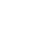• 全部
• 经验案例
• 典型配置
• 技术公告
• FAQ
• 全部
• 全部
 产品线 搜索 取消 案例类型 发布者 是否解决 是否官方 时间

# 出口交换机

2019-07-12提问
• 0关注
• 1收藏，477浏览

#### 问题描述：

C:\Users\wzp>ping 10.46.10.254 -t 正在 Ping 10.46.10.254 具有 32 字节的数据: 来自 10.46.10.254 的回复: 字节=32 时间=56ms TTL=255 来自 10.46.10.254 的回复: 字节=32 时间=86ms TTL=255 来自 10.46.10.254 的回复: 字节=32 时间=32ms TTL=255 来自 10.46.10.254 的回复: 字节=32 时间=14ms TTL=255 来自 10.46.10.254 的回复: 字节=32 时间=8ms TTL=255 来自 10.46.10.254 的回复: 字节=32 时间=7ms TTL=255 来自 10.46.10.254 的回复: 字节=32 时间=2ms TTL=255 来自 10.46.10.254 的回复: 字节=32 时间=9ms TTL=255 来自 10.46.10.254 的回复: 字节=32 时间=13ms TTL=255 来自 10.46.10.254 的回复: 字节=32 时间=1ms TTL=255 来自 10.46.10.254 的回复: 字节=32 时间=4ms TTL=255 来自 10.46.10.254 的回复: 字节=32 时间=5ms TTL=255 来自 10.46.10.254 的回复: 字节=32 时间=20ms TTL=255 来自 10.46.10.254 的回复: 字节=32 时间=13ms TTL=255 来自 10.46.10.254 的回复: 字节=32 时间=2ms TTL=255 来自 10.46.10.254 的回复: 字节=32 时间=6ms TTL=255 来自 10.46.10.254 的回复: 字节=32 时间=3ms TTL=255

3 个回答

## 提出建议

+×

×

×

• 1. 您认为哪些内容泄露了您的隐私？（请在邮件中列出您举报的内容、链接地址，并给出简短的说明）
• 2. 您是谁？（身份证明材料，可以是身份证或护照等证件）

×

• 1. 您举报的内容是什么？（请在邮件中列出您举报的内容和链接地址）
• 2. 您是谁？（身份证明材料，可以是身份证或护照等证件）
• 3. 是哪家企业？（营业执照，单位登记证明等证件）
• 4. 您与该企业的关系是？（您是企业法人或被授权人，需提供企业委托授权书）

×

×

• 1. 您举报的内容以及侵犯了您什么权益？（请在邮件中列出您举报的内容、链接地址，并给出简短的说明）
• 2. 您是谁？（身份证明材料，可以是身份证或护照等证件）

×

×